# Drawing Quadratic and Cubic Graphs Math Review

math tutorials > drawing quadratic and cubic graphs

The plotting of quadratic and cubic graphs is a vast topic and can be done in various ways.  The aim of this tutorial is to introduce you to the 2 types of graphs and the tabular method of drawing them (as we did for straight line graphs).

The equation for a quadratic graph can be written as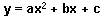a, b, and c are constants.
y has the power of 1.
x has a maximum power of 2.

The graphs of quadratic equations are called parabolas.

### Examples

These are all parabolas: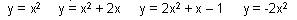### Drawing the graph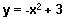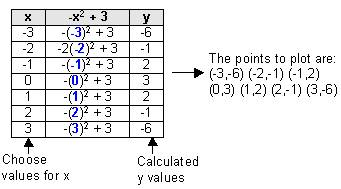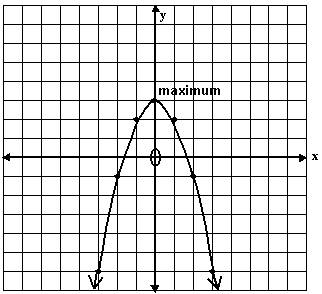## Parabola problems for you to try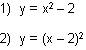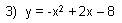## Cubic Graphs.

Cubic graphs are in the form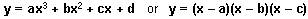a, b, c and d are constants.
y has a power of 1.
x has a maximum power of 3.

### Examples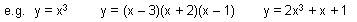###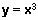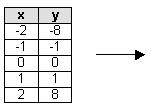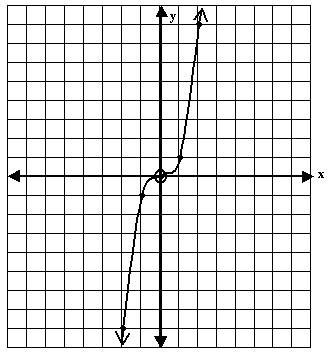## Cubic graph problems for you to try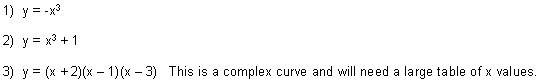### Note:

You can calculate the intercepts of parabolas and cubics in the same way as for straight lines.
For the x-intercept, put y = 0.  (A parabola may have 2 x-intercepts, a cubic 3).
For the y-intercept, put x = 0.